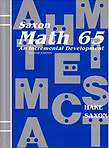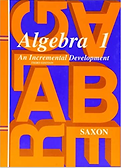# Courses

Assessments are required to ensure proper class placement. Interview required for 11 year old scholar consideration.

### Fundamentals of MathematicsInstructor:  Gesse Rosa

Course Description:

The topics covered in this course include: solving numeric expressions with whole number and simple fractions; solving algebraic expressions of single variable, long division, multiplication, comparisons, etc. Our goal will be to complete four lessons from the text each week.Duration: 30 weeks, four lessons per week

Expected Homework: 30-60 minutes a day, one lesson per day

Coursework Location: Google Classroom (Syllabus, homework assignments, instructional videos).

Parents need to know username and password to monitor homework progress. Please encourage daily homework and inform instructor if falling behind.

Text: Math 65: An Incremental Development 2nd Edition, Saxon   ISBN: 9781565770362

### Pre-Algebra 1Instructor: Gesse Rosa

Prerequisite: Mastery of Fundamentals of Mathematics or equivalent.

Course Description:

The concepts studied in this course include numeration, basic operations, fractions, decimals, percentages, ratios, rates, estimation, and number theory. The textbook for this class is Math 76, third edition, Saxon. Four lessons will be taught each week. Students will demonstrate their learning of the math concepts through in-class discussion and daily homework.Duration: 30 weeks, four lessons per week

Expected Homework: 30-60 minutes a day, one lesson per day

Coursework Location: Google Classroom (Syllabus, homework assignments, instructional videos).

Parents need to know username and password to monitor homework progress. Please encourage daily homework and inform instructor if falling behind.

Text: Math 76: An Incremental Development 3rd Edition, Saxon   ISBN: 978-1565771536

### Algebra 1Instructor: Gesse Rosa

Prerequisite: Mastery of the Pre-Algebra or equivalent.

Course Description:​

Some of the main math concepts explored in this course include the following: evaluation of numeric and algebraic expressions, exponents and roots, properties of the real numbers, absolute value and equations and inequalities involving absolute value, scientific notation, unit conversions; solution of equations of one variable, solution of system of linear equations, the algebra of polynomials and rational expressions, word problems requiring algebra for their solution, graphical solution of simultaneous equations. The pythagorean theorem, algebraic proofs, functions and functional notation, solution of quadratic equations via factoring and completing the square, direct and inverse variation, and exponential growth, computation of the perimeter and areas of two-dimensional regions, computation of the surface area and volume of a wide variety of geometric solids, and statistics and probability will also be explored.Duration: 30 weeks, four lessons per week

Expected Homework: 30-60 minutes a day, one lesson per day

Coursework Location: Google Classroom (Syllabus, homework assignments, instructional videos).

Parents need to know username and password to monitor homework progress. Please encourage daily homework and inform instructor if falling behind.

Text: Algebra 1: An Incremental Development, 3rd Edition, Saxon    ISBN: 978-1565771345

### Algebra 2Instructor: Gesse Rosa

Prerequisite: Mastery of Algebra I

Course Description​:

The concepts explored in Algebra 2  include the following: graphical solution to simultaneous equations, scientific notation, radicals, roots of quadratic equations including complex roots, properties of the real numbers, inequalities and systems of inequalities, logarithms and antilogarithms, exponential equations, basic trigonometric functions, algebra of polynomials, vectors, polar and rectangular coordinate systems, and a wide spectrum of word problems requiring algebra to solve. Algebra 2 provide the student with a solid understanding of geometry. A few exercises involving concepts of physics and chemistry are included in the course (gas law, force vector, chemical mixture, percent markups, etc). Set theory, probability and statistics, and other topics are also treated. The most reliable way to receive specific information about course work, including topics and timing, is on-line. For many students, the most challenging part of class is to apply concepts through story problems and signed (negative) number operations.Duration: 30 weeks, four lessons per week

Expected Homework: 30-60 minutes a day, one lesson per day

Coursework Location: Google Classroom (Syllabus, homework assignments, instructional videos).

Parents need to know username and password to monitor homework progress. Please encourage daily homework and inform instructor if falling behind.

Text: Algebra 2: An Incremental Development, 3rd Edition, Saxon    ISBN: 978-1565771406

### Geometry/TrigonometryInstructor: Gesse Rosa

Prerequisite: Pre-algebra

Course Description

The topics covered in this course include: construction of angles, parallel and perpendicular lines, properties of geometric shapes, the geometry of right triangles, interior and exterior angles. transformation on planar geometric shapes, definition of the six trigonometric functions, Pythagoras theorem. This course is a solid introduction to geometry and trigonometry. It will be a great preparation for mastering the geometry and trigonometry portion of ACT.

Duration: 30 weeks, four lessons per week

Expected Homework: 30-60 minutes a day, one lesson per day

Coursework Location: Google Classroom (Syllabus, homework assignments, instructional videos)

Parents need to know username and password to monitor homework progress. Please encourage daily homework and inform instructor if falling behind.

Text: No textbook for this class. All material will be provided by the instructor.

### General Chemistry 1Instructor: Gesse Rosa

Course Description

This course is the first part of general chemistry. The topics covered includes the following: Analyzing data, properties of matter, structure of the atom, electron configuration, understanding the periodic table, ionic compounds, covalent compounds, chemical reactions, the concept of mole, stoichiometry, and gases. The textbook for this class is Chemistry, Matter and Change, Glencoe Science. This course will study the first 12 chapters of the textbook. This class will help prepare scholar for science portion of ACTs.Duration: 30 weeks, first 12 chapters of the book

Expected Homework: 30-60 minutes a day

Coursework Location: Google Classroom (Syllabus, homework assignments, instructional videos)

Parents need to know username and password to monitor homework progress. Please encourage daily homework and inform instructor if falling behind.

Text: Chemistry: Matter & Change, Student Edition (Glencoe Science)     ISBN-13: 978-0078746376

### Fundamentals of ChemistryInstructor: Gesse Rosa

Course Description

This course is for students who have never studied chemistry. The topics explored includes: Matter, properties of matter, density,  and understanding the Periodic Table of the elements. Students also will study concepts of atomic mass and number.  After the conclusion of this course, the student will have solid understanding of Periodic Table of the elements.

Duration: 30 weeks

Expected Homework: only class work.

Coursework Location: Google Classroom (Syllabus, homework assignments, instructional videos)

Text: No text, course work provided by instructor.

### ACT Prep Instructor: Gesse Rosa

Prerequisite: Mastery of the Algebra I or registered for Algebra 1

Course Description

This class focus on the math and science portion of the ACT. The goal is to review the concepts of a ACT exam each month. All problems will be from ACT past tests of math and science.

Duration: 30 weeks, one week will focus on math and the next week will focus on science and so on

Coursework Location: Google Classroom (Syllabus, homework assignments, instructional videos)

Parents need to know username and password to monitor homework progress. Please encourage daily homework and inform instructor if falling behind.

Text: No text, course work provided by instructor.